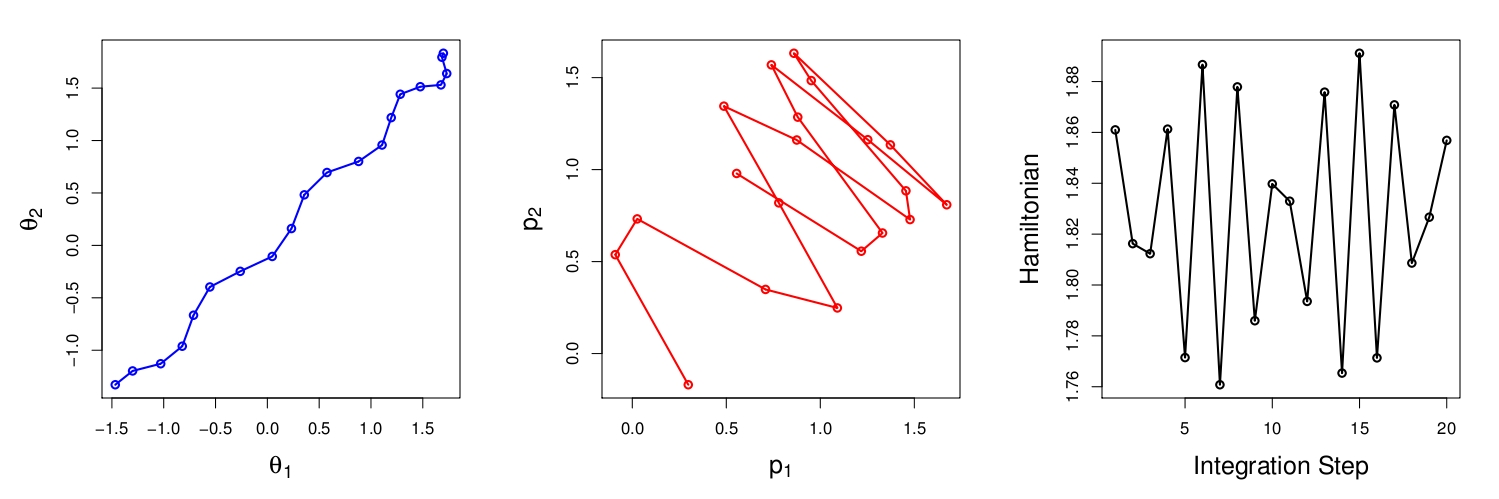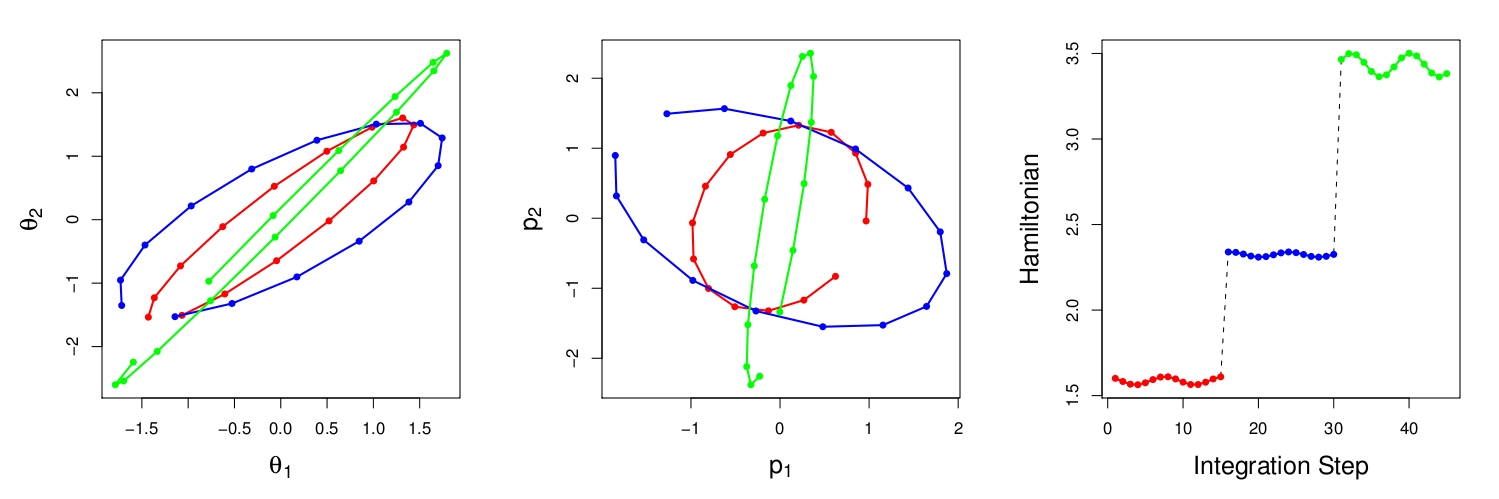Want to share your content on R-bloggers? click here if you have a blog, or here if you don't.

Here is a (prompt!) reply from Mark Girolami corresponding to the earlier post:

In preparation for the Read Paper session next month at the RSS, our research group at CREST has collectively read the Girolami and Calderhead paper on Riemann manifold Langevin and Hamiltonian Monte Carlo methods and I hope we will again produce a joint arXiv preprint out of our comments. (The above picture is reproduced from Radford Neal’s talk at JSM 1999 in Baltimore, talk that I remember attending!) Although this only represents my preliminary/early impression on the paper, I have trouble with the Physics connection. Because it involves continuous time events that are not transcribed directly into the simulation process.It might be easier to look at the two attached figures rather than the isocontours in the complete phase space (q, p) of the 1-d Gaussian that is in the pic you include in your post. Both figures relate to sampling from a zero mean bivariate Gaussian with covariance matrix$Sigma$ — marginal variance 1 and cross-covariance$rho = 0.6$ (example taken from Neal 2010). In the first figure there are three panes showing the 20 integration steps obtained by standard HMC where the metric tensor for the space of bivariate Gaussians is NOT employed and so the leftmost plot shows the proposal effectively moving from -1.5, -1 to 1.5, 1.5 basically a large traversal over the 2d sampling space. The middle plot shows the corresponding momentum variable steps and the right plot shows the total Hamiltonian value (energy or negative joint likelihood) where the difference at the start and end of the integration is very small indicating a high probability of acceptance. That is all fine – large proposal step accepted with high probability.Now lets look at the second figure. Lay aside the Physics interpretation and let’s try to adopt a geometric one in this case to see if this helps the understanding. So our task is to simulate from this bivariate Gaussian we know that the metric tensor$Sigma^{-1}$ defines a flat manifold of bivariate densities. Now if we wanted to move from one point to another we would wish to take the most direct route – the shortest path in the space — the geodesic in other words. The geodesics on a manifold are defined by the second order differential equation in terms of the coordinates (our sample space) and the Christofell symbols of the manifold — so to make a proposed move along the geodesic we need to solve the differential equations describing the geodesic. Now by rewriting the geodesic equation in a different form we end up with Hamiltons equations -— in other words the solution flow of the geodesic equations coincides with the Hamiltonian flows — so solving Hamiltons equations is just another way to solve the geodesic equation. So the variable p just emerges from the rewriting of the geodesic equation and nothing more. In this case as the manifold corresponding to bivariate Gaussians is flat and the metric tensor is the inverse of the covariance matrix — the geodesics will be elliptical paths defined by$Sigma$ — in other words the isocontours of the bivariate Gaussian. So the first panel in this figure shows 15 integration steps and as can be observed the path follows the elliptical isocontour of the target density — as the p variable is the dual in the geodesic equations then it also maps out an isocontour defined by the metric tensor.

Does that alternative explanation of the deterministic proposal mechanism help?? If we accept the geometric view and wish to make proposals that follow the geodesics then integrating Hamiltons equations is just another way to solve the geodesic equations nothing more — so there is no need to appeal to the Physics interpretation at all — I am considering actually presenting the method from that perspective at the RSS meeting — thoughts on that?

Overall, trying to take advantage of second order properties of the target, just like the Langevin improvement takes advantage of the first order?is a natural idea which, when implementable, can obviously speed up convergence. This is the Langevin part, which may use a fixed metric M or a local metric defining a Riemann manifold, G(.). So far, so good, assuming the derivation of an observed or expected information G(.) is feasible up to some approximation level. The Hamiltonian part that confuses me introduces a dynamic on level sets of where p is an auxiliary vector of dimension D. Namely. while I understand the purpose of the auxiliary vector, namely to speed up the exploration of the posterior surface by taking advantage of the additional energy provided by p, I fail to understand why the fact that the discretised (Euler) approximation to Hamilton?s equations is not available in closed form is such an issue?. The fact that the (deterministic?) leapfrog integrator is not exact should not matter since this can be corrected by a Metropolis-Hastings step.

I think that I have attempted to answer your question about the auxilliary vector p. So the leapfrog integrator is NOT exact — it does not EXACTLY preserve the Hamiltonian — it is second order accurate — and the MH step corrects for the bias in the invariant measure introduced by this error.

The integrator does exactly preserve volume elements — so the determinant is not required to appear in the MH ratio — of course this requirement could be relaxed and then we would have to include the Jacobian in the MH ratio — this might be ok to compute. It is also symmetric/reversible so the effective proposal densities do not need to appear in the MH ratio – just the ratio of the joint likelihoods (q and p). In this paper I wanted to make sure everything was exact and formally correct and let further work relax these restrictions and develop more efficient numerics and / or approximations.

While the logistic example is mostly a toy problem (where importance sampling works extremely well, as shown in our survey with Jean-Michel Marin), the stochastic volatility is more challenging and the fact that the Hamiltonian scheme applies to the missing data (volatility) as well as to the three parameters of the model is quite interesting. I however wonder at the appeal of this involved scheme when considering that the full conditional of the volatility can be simulated exactly?

The LR example is indeed a toy one and it was used just to calibrate and illustrate the various methods with other well known methods — with the SV as with the log-Cox model we chose to sample from the conditionals of latent volatilities given parameters and then parameters given volatilities -—in a Gibbs style scheme — we coud also have sampled jointly or used the exact sampling from the conditional (which I must confess I had not noticed at the time) — the point being that these are illustrative of the methods proposed — I made no claim to optimality of the schemes used — they were used to illustrate the potential of the methodology.

Reversibility is a “second-order” property for MCMC algorithms. E.g., the Gibbs sampler is not reversible but does work without that property? In addition, the standard Metropolis-Hastings scheme is reversible by construction (courtesy of the detailed balance property). My point in that post (and in the incoming discussion) is that, similar to Langevin, the continuous time “analogy” may be unnecessary costly in trying to carry this analogy in discrete (algorithmic) time. We are not working in continuous time, the invariance/stability properties of the continuous time process are not carried on to the discretised version of the process, we therefore should not care about reproducing exactly the continuous time process in discrete time. For instance, when considering the Langevin diffusion, the corresponding Langevin algorithm could use another scale for the gradient than the one used for the noise, i.e.rather than the Euler discretisation. A few experiments at the time of the first edition of MCSM (Chapter 6, Section 6.5) showed that a different scale could lead to improvements.

Correct — again I wanted to be ‘purer than pure’ in the way this work was presented and whilst indeed different scaling for the drift and the diffusion terms in the Langevin scheme can work and imporve things — I felt that this is follow on work from the ideas presented — for example there are other discretisations of the Langevin SDE that may be more efficient than a simple first order Euler – which I do discuss in the final section.Filed under: R, Statistics, University life Tagged: Euler approximationm Hamiltonian, geodesics, JRSSB, London, Read paper, RSS